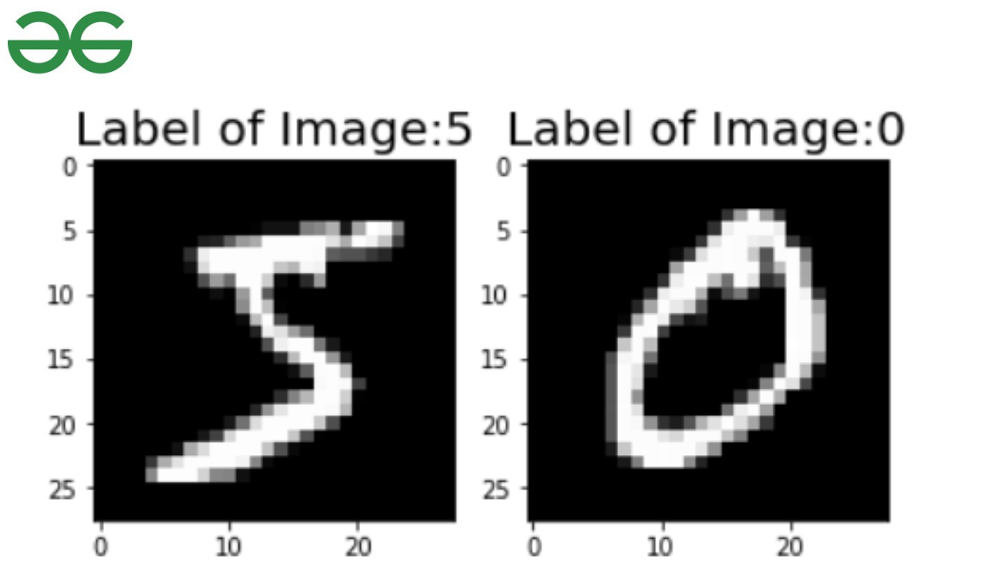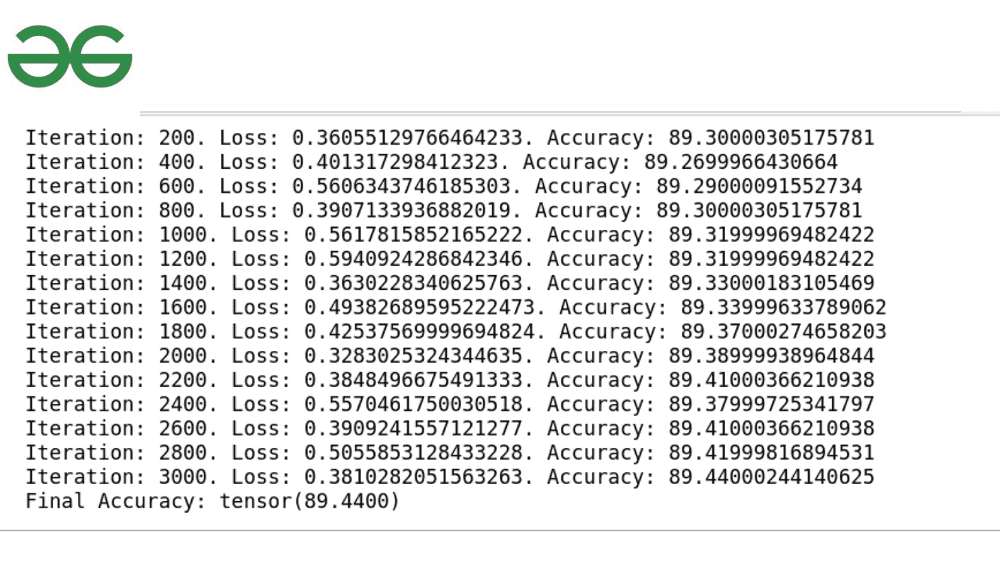Open in App
Not now

# Logistic Regression on MNIST with PyTorch

• Difficulty Level : Medium
• Last Updated : 28 Mar, 2022

## Logistic Regression

Logistic Regression is also known as Binary Classification is one of the most popular Machine Learning Algorithms. It comes under Supervised Learning Classification Algorithms. It is used to predict the probability of the target label. By binary classification, it means that the model predicts the label either 0 or 1. The target variable is categorical data types for example: Yes or No, Survived or Not Survived, Male or Female, Pass or Fail, and so on. Logistic Regression makes use of the Sigmoid Function to make the prediction. Sigmoid Activation Function is a nonlinear function which is defined as:

```y = 1/(1+e-z)

#the y is in range 0-1
#z = x*w + b where w is weight and b is bias```

## Logistics Regression of MNIST In Pytorch

Pytorch is the powerful Machine Learning Python Framework. With the Pytorch framework, it becomes easier to implement Logistic Regression and it also provides the MNIST dataset.

Installation:

```pip install torch
pip install torchvision --no-deps```

### Import Necessary Modules

```import torch
import torchvision
import torch.nn as tn
import matplotlib.pyplot as plt
import torchvision.transforms as tt
import torch.utils as utils```

Torchvision module provides the MNIST dataset which can be downloaded by entering this code:

## Python

 `train_data ``=` `torchvision.datasets.MNIST(``'./data'``,download``=``True``) ` `test_data ``=` `torchvision.datasets.MNIST(``'data'``,train``=``False``) ` `print``(train_data) ` `print``(test_data)`

Output:

```Dataset MNIST
Number of datapoints: 60000
Root location: ./data
Split: Train
Dataset MNIST
Number of datapoints: 10000
Root location: data
Split: Test```

Ok, the training data and test data that we have now are in form of Images:

## Python3

 `print``(train_data[``0``])`

Output:

`(<PIL.Image.Image image mode=L size=28x28 at 0x7FEFA4362E80>, 5)`

If you notice the output carefully we get an insight into the train data. The image is made of 28*28 pixels and the first index image is 5. Since it is in form of a tuple where index one is the image and index two is the Code block label. Using Matplotlib we can visualize what image does first train data index contains.

## Python3

 `import` `matplotlib.pyplot as plt ` ` `  `plt.subplot(``1``,``2``,``1``) ` `image, label ``=` `train_data[``0``] ` `plt.imshow(image, cmap``=``'gray'``) ` `plt.title(``"Label of Image:{}"``.``format``(label),fontsize``=``20``) ` `plt.subplot(``1``,``2``,``2``) ` `image, label ``=` `train_data[``1``] ` `plt.imshow(image, cmap``=``'gray'``) ` `plt.title(``"Label of Image:{}"``.``format``(label),fontsize``=``20``)`

#### Output:### Data Transformation:

Since the data is in image form, it has to be transformed into Tensor, so that PyTorch neural network can train the data. Torchvision provides a transform method.

```train_data = torchvision.datasets.MNIST('data',train=True,transform=tt.ToTensor())
test_data = torchvision.datasets.MNIST('data',train=False,transform=tt.ToTensor()) ```

#### Arguments Required

input_size = 28*28  #Size of image

num_classes = 10  #the image number are in range 0-10

num_epochs = 5 #one cycle through the full train data

batch_size = 100 #sample size consider before updating the model’s weights

learning_rate = 0.001  #step size to update parameter

#### Make It Iterable

Using DataLoader we can loop through the tensor of the train data.

```train_dataLoader = torch.utils.data.DataLoader(train_data, batch_size=batch_size,shuffle=True)

### Build Logistic Regression Model

Create a model of Logistic Regression that fits in features and output data.

```class LogisticRegression(tn.Module):
def __init__(self,input_size,num_classes):
super(LogisticRegression,self).__init__()
self.linear = tn.Linear(input_size,num_classes)

def forward(self,feature):
output = self.linear(feature)
return output```

### Loss And Optimizer

We shall use the CrossEntropyLoss function to calculate the computed loss. The reason to use the CrossEntropyLoss function is that it computes the softmax function and cross-entropy.

And Stochastic Gradient Descent is the optimizer used to calculate the gradient and update the parameters.

```model = LogisticRegression(input_size,num_classes)
loss = tn.CrossEntropyLoss()
optimizer = torch.optim.SGD(model.parameters(),lr=learning_rate)```

### Train And Predict

Convert images and labels to tensors with gradients and then clear gradients w.r.t parameters(optimizer.zero_grad()). Compute the loss from the gradient and further try to reduce the loss by updating the parameters by adding a learning rate. This process is called Backward Propagation.

```run = 0
for epoch in range(num_epochs):

#forward propagation
output = model(images)
# compute loss based on obtained value and actual label
compute_loss = loss(output,labels)
# backward propagation
compute_loss.backward()
# update the parameters
optimizer.step()
run+=1

if (i+1)%200 == 0:
# check total accuracy of predicted value and actual label
accurate = 0
total = 0
output = model(images)
_,predicted = torch.max(output.data, 1)
# total labels
total+= labels.size(0)

# Total correct predictions
accurate+= (predicted == labels).sum()
accuracy_score = 100 * accurate/total

print('Iteration: {}. Loss: {}. Accuracy: {}'.format(run, compute_loss.item(), accuracy_score))

print('Final Accuracy:',accuracy_score)```

### Final Result:The accuracy score is 89, which is not bad.

My Personal Notes arrow_drop_up
Related Articles